# RD Sharma Solutions for Class 6 Maths Chapter 12: Triangles Exercise 12.1

The students would have learnt about line segments, angles, rays and lines in the previous chapters. A plane figure which is formed by three non-parallel line segments is called a Triangle. In Exercise 12.1, the students learn about triangles and their types using various methods of classification. The students can download RD Sharma Solutions Class 6 Maths Chapter 12 Triangles Exercise 12.1 PDF, which is a vital resource of study material to boost exam preparation.

## RD Sharma Solutions for Class 6 Maths Chapter 12: Triangles Exercise 12.1 Download PDF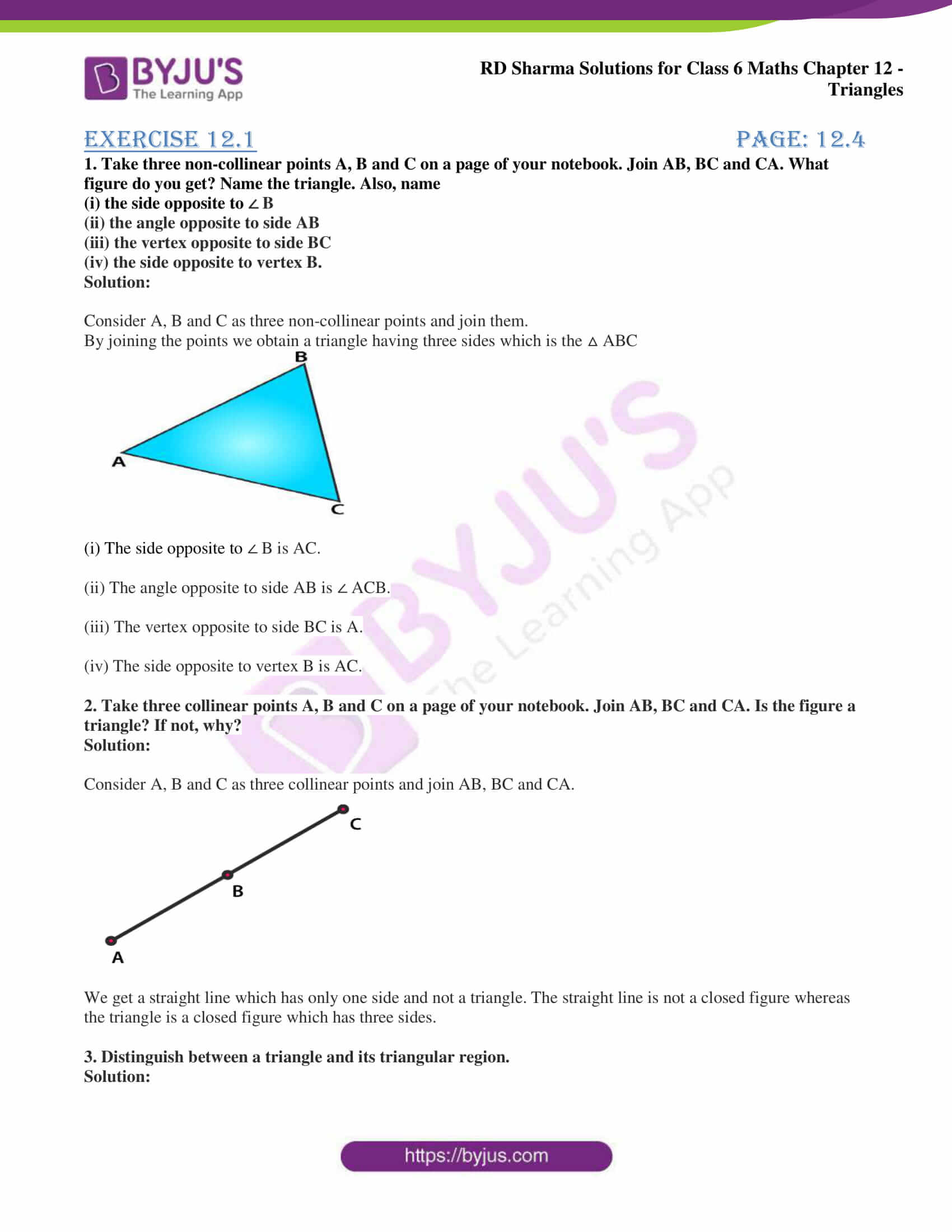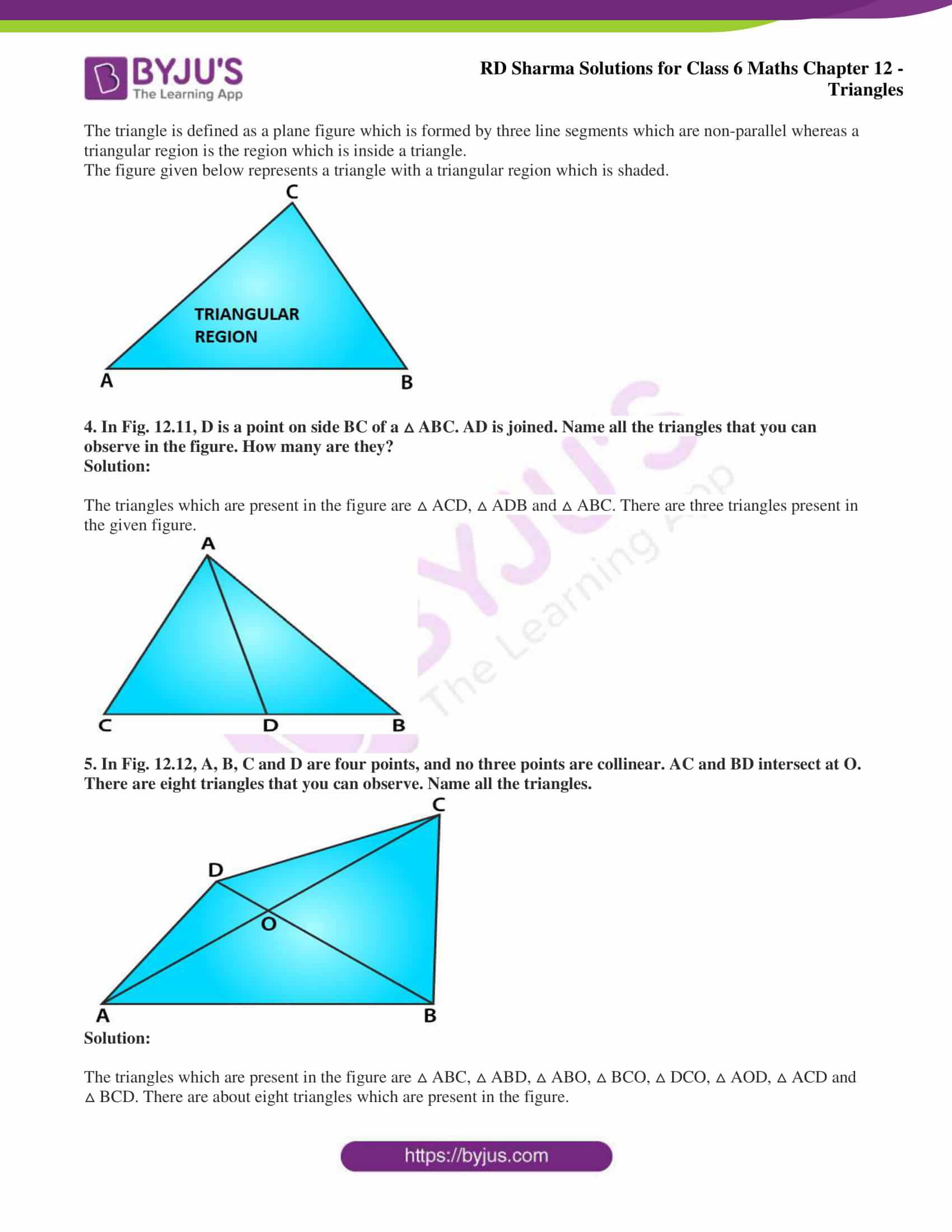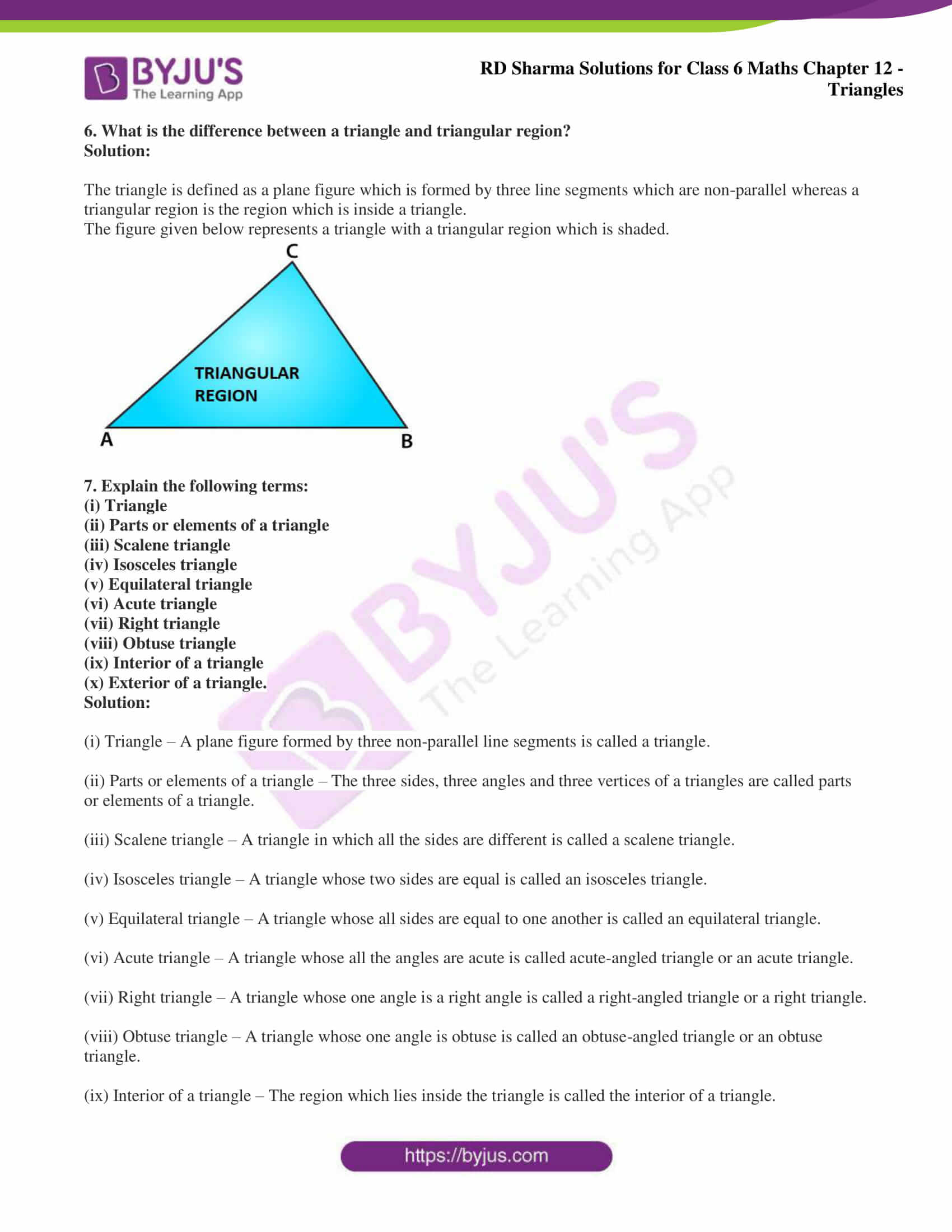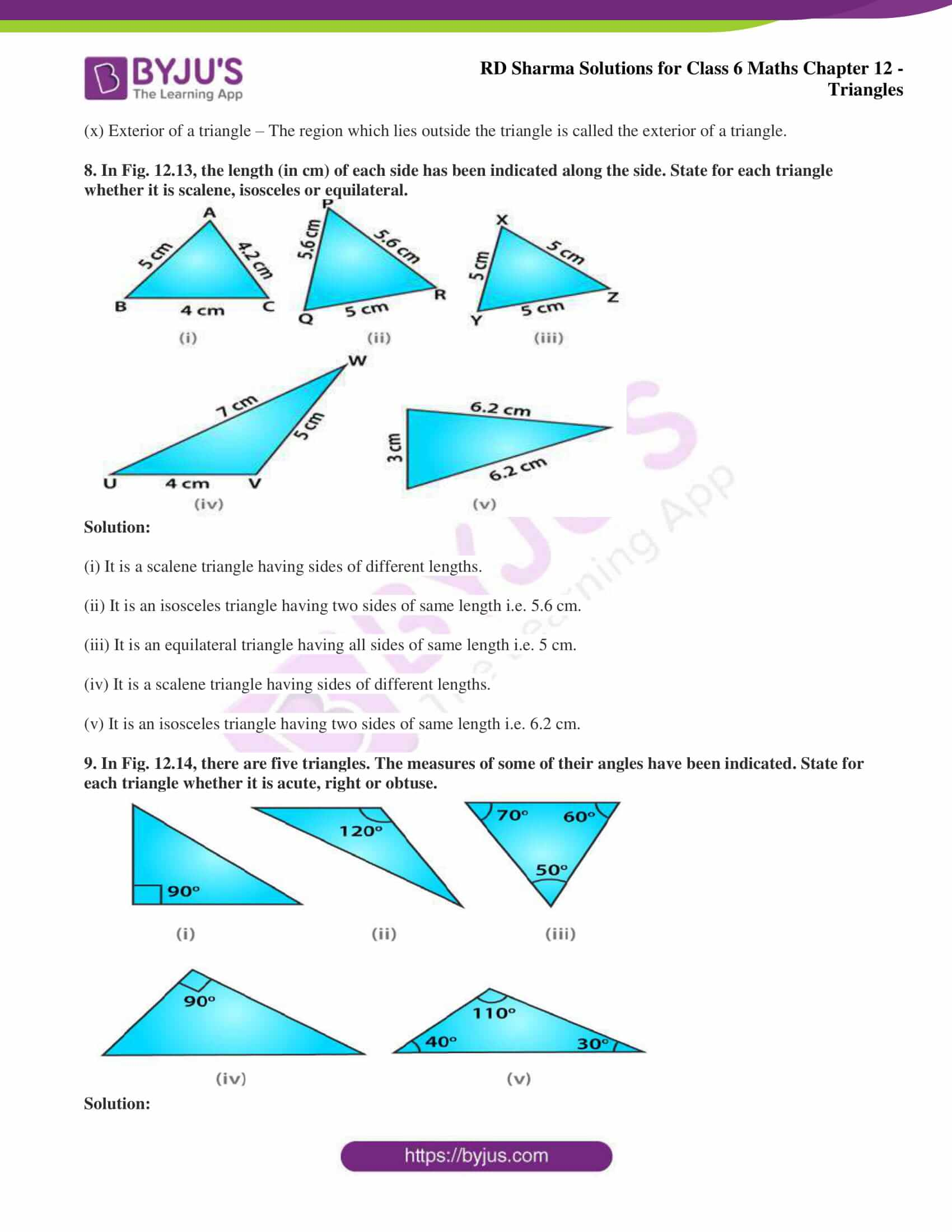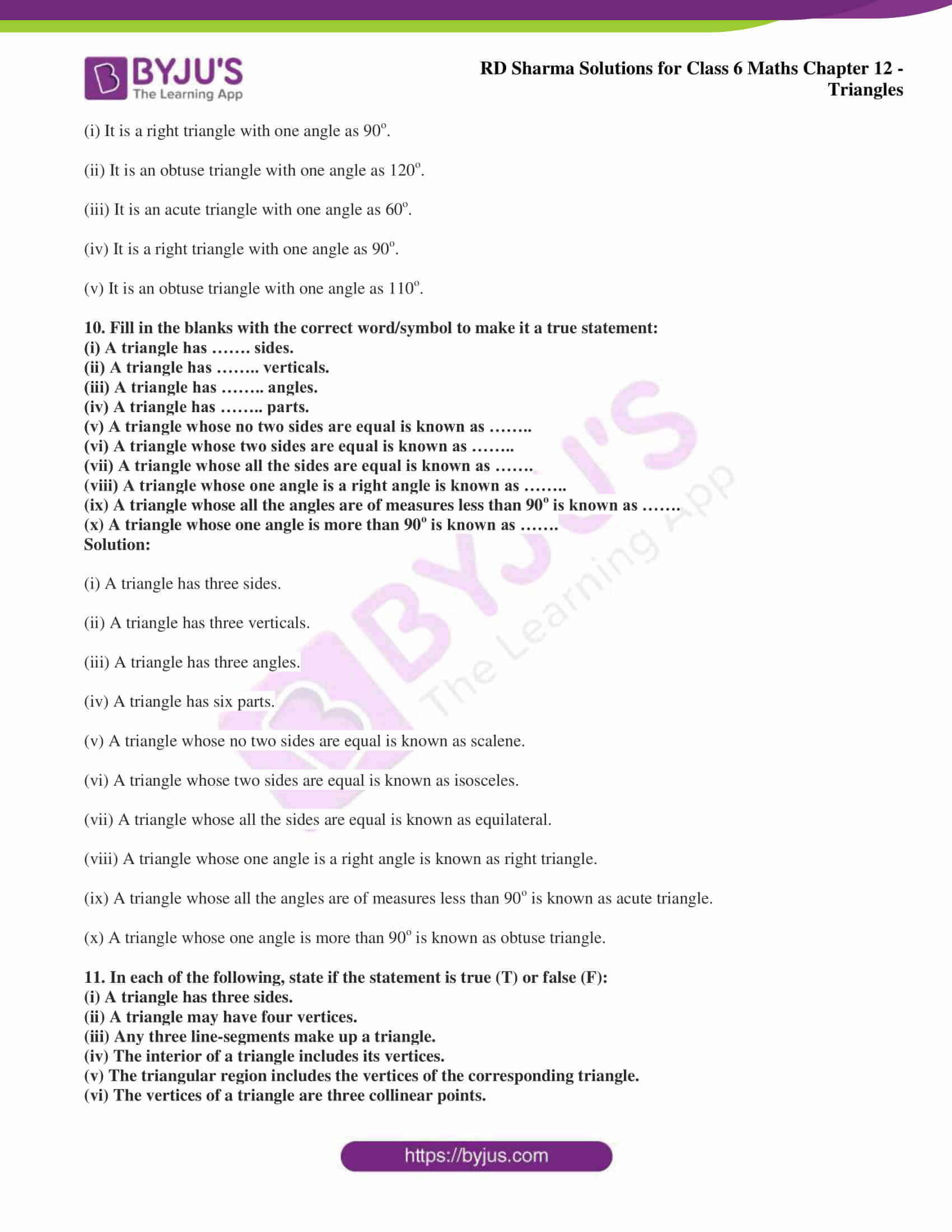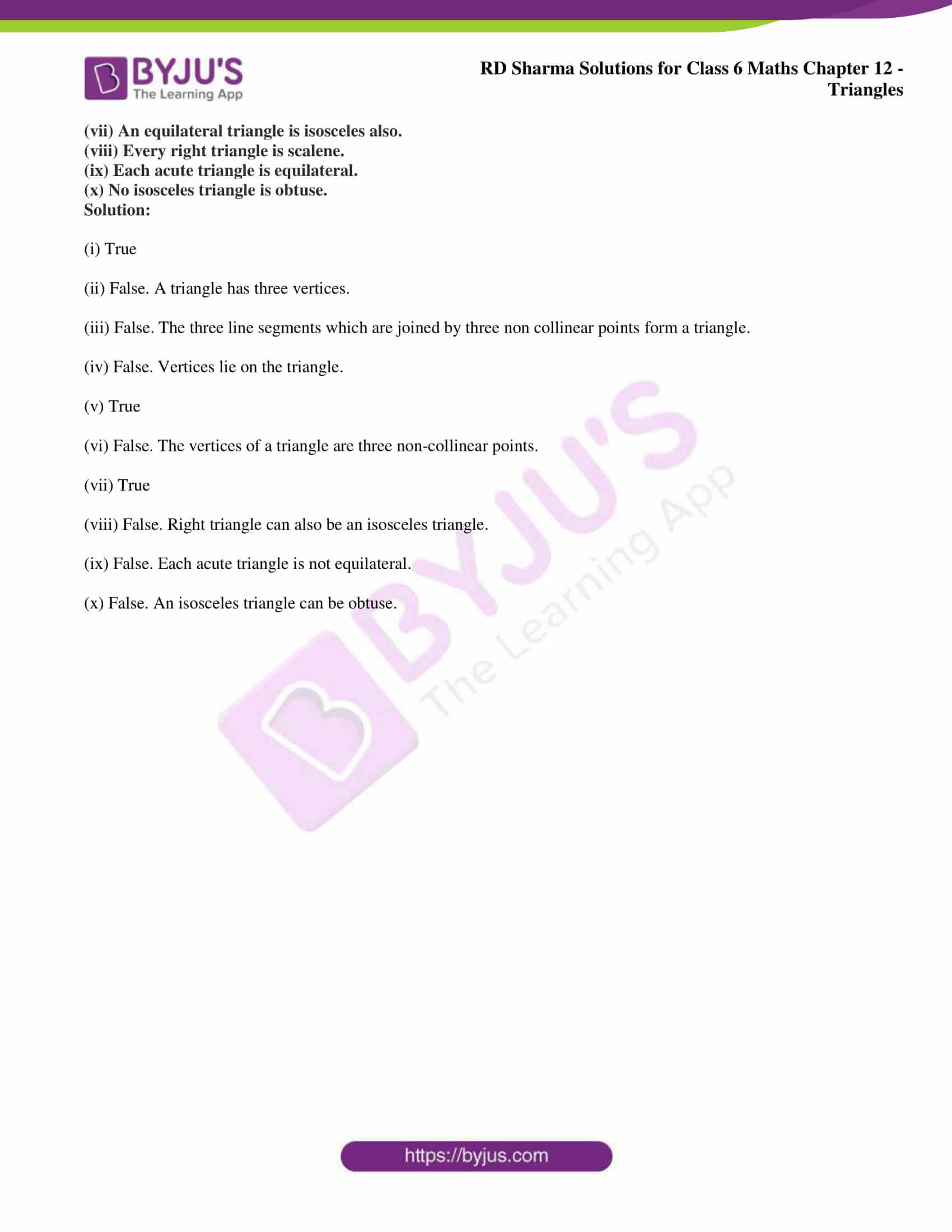### Access answers to Maths RD Sharma Solutions for Class 6 Chapter 12: Triangles Exercise 12.1

1. Take three non-collinear points A, B and C on a page of your notebook. Join AB, BC and CA. What figure do you get? Name the triangle. Also, name

(i) the side opposite to ∠ B

(ii) the angle opposite to side AB

(iii) the vertex opposite to side BC

(iv) the side opposite to vertex B.

Solution:

Consider A, B and C as three non-collinear points and join them.

By joining the points we obtain a triangle having three sides which is the △ ABC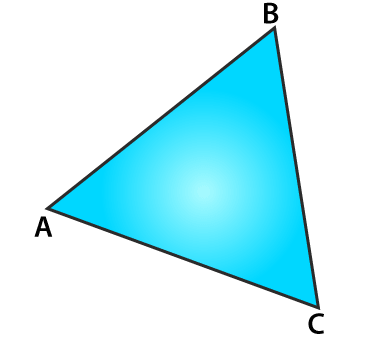(i) The side opposite to ∠ B is AC.

(ii) The angle opposite to side AB is ∠ ACB.

(iii) The vertex opposite to side BC is A.

(iv) The side opposite to vertex B is AC.

2. Take three collinear points A, B and C on a page of your notebook. Join AB, BC and CA. Is the figure a triangle? If not, why?

Solution:

Consider A, B and C as three collinear points and join AB, BC and CA.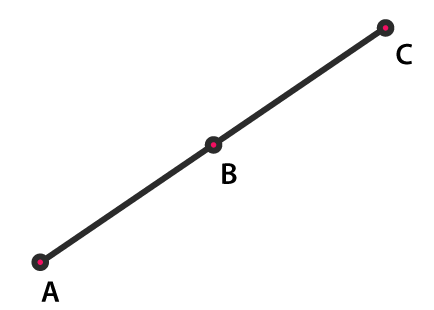We get a straight line which has only one side and not a triangle. The straight line is not a closed figure whereas the triangle is a closed figure which has three sides.

3. Distinguish between a triangle and its triangular region.

Solution:

The triangle is defined as a plane figure which is formed by three line segments which are non-parallel whereas a triangular region is the region which is inside a triangle.

The figure given below represents a triangle with a triangular region which is shaded.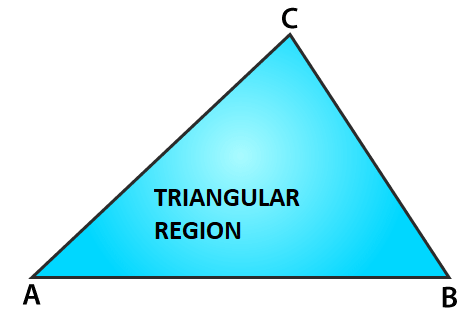4. In Fig. 12.11, D is a point on side BC of a △ ABC. AD is joined. Name all the triangles that you can observe in the figure. How many are they?

Solution:

The triangles which are present in the figure are △ ACD, △ ADB and △ ABC. There are three triangles present in the given figure.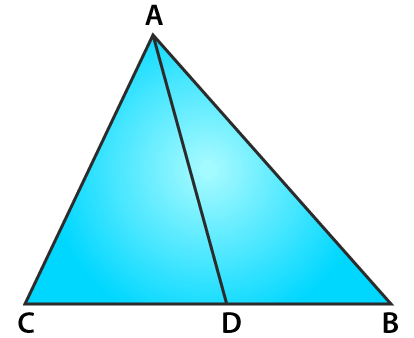5. In Fig. 12.12, A, B, C and D are four points, and no three points are collinear. AC and BD intersect at O. There are eight triangles that you can observe. Name all the triangles.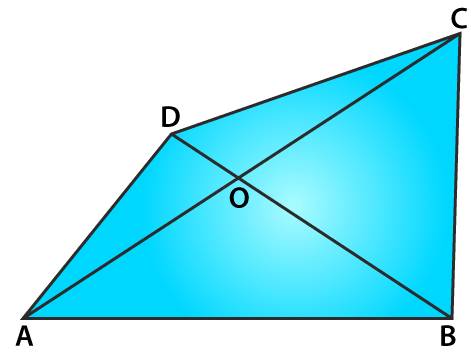Solution:

The triangles which are present in the figure are △ ABC, △ ABD, △ ABO, △ BCO, △ DCO, △ AOD, △ ACD and △ BCD. There are about eight triangles which are present in the figure.

6. What is the difference between a triangle and triangular region?

Solution:

The triangle is defined as a plane figure which is formed by three line segments which are non-parallel whereas a triangular region is the region which is inside a triangle.

The figure given below represents a triangle with a triangular region which is shaded.7. Explain the following terms:

(i) Triangle

(ii) Parts or elements of a triangle

(iii) Scalene triangle

(iv) Isosceles triangle

(v) Equilateral triangle

(vi) Acute triangle

(vii) Right triangle

(viii) Obtuse triangle

(ix) Interior of a triangle

(x) Exterior of a triangle.

Solution:

(i) Triangle – A plane figure formed by three non-parallel line segments is called a triangle.

(ii) Parts or elements of a triangle – The three sides, three angles and three vertices of a triangles are called parts or elements of a triangle.

(iii) Scalene triangle – A triangle in which all the sides are different is called a scalene triangle.

(iv) Isosceles triangle – A triangle whose two sides are equal is called an isosceles triangle.

(v) Equilateral triangle – A triangle whose all sides are equal to one another is called an equilateral triangle.

(vi) Acute triangle – A triangle whose all the angles are acute is called acute-angled triangle or an acute triangle.

(vii) Right triangle – A triangle whose one angle is a right angle is called a right-angled triangle or a right triangle.

(viii) Obtuse triangle – A triangle whose one angle is obtuse is called an obtuse-angled triangle or an obtuse triangle.

(ix) Interior of a triangle – The region which lies inside the triangle is called the interior of a triangle.

(x) Exterior of a triangle – The region which lies outside the triangle is called the exterior of a triangle.

8. In Fig. 12.13, the length (in cm) of each side has been indicated along the side. State for each triangle whether it is scalene, isosceles or equilateral.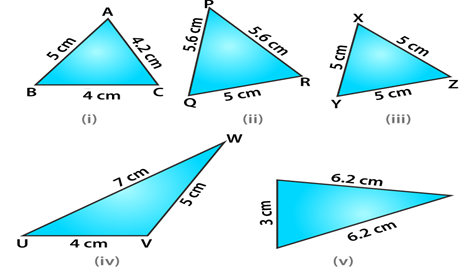Solution:

(i) It is a scalene triangle having sides of different lengths.

(ii) It is an isosceles triangle having two sides of same length i.e. 5.6 cm.

(iii) It is an equilateral triangle having all sides of same length i.e. 5 cm.

(iv) It is a scalene triangle having sides of different lengths.

(v) It is an isosceles triangle having two sides of same length i.e. 6.2 cm.

9. In Fig. 12.14, there are five triangles. The measures of some of their angles have been indicated. State for each triangle whether it is acute, right or obtuse.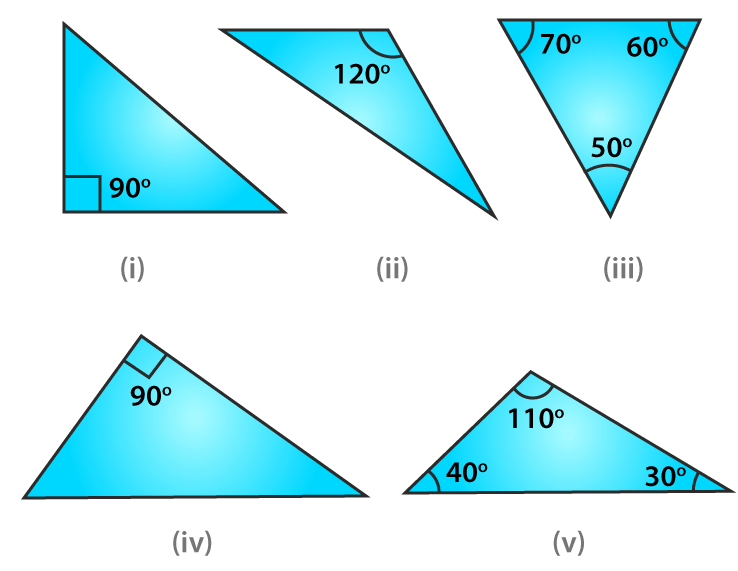Solution:

(i) It is a right triangle with one angle as 90o.

(ii) It is an obtuse triangle with one angle as 120o.

(iii) It is an acute triangle with one angle as 60o.

(iv) It is a right triangle with one angle as 90o.

(v) It is an obtuse triangle with one angle as 110o.

10. Fill in the blanks with the correct word/symbol to make it a true statement:

(i) A triangle has ……. sides.

(ii) A triangle has …….. verticals.

(iii) A triangle has …….. angles.

(iv) A triangle has …….. parts.

(v) A triangle whose no two sides are equal is known as ……..

(vi) A triangle whose two sides are equal is known as ……..

(vii) A triangle whose all the sides are equal is known as …….

(viii) A triangle whose one angle is a right angle is known as ……..

(ix) A triangle whose all the angles are of measures less than 90o is known as …….

(x) A triangle whose one angle is more than 90o is known as …….

Solution:

(i) A triangle has three sides.

(ii) A triangle has three verticals.

(iii) A triangle has three angles.

(iv) A triangle has six parts.

(v) A triangle whose no two sides are equal is known as scalene.

(vi) A triangle whose two sides are equal is known as isosceles.

(vii) A triangle whose all the sides are equal is known as equilateral.

(viii) A triangle whose one angle is a right angle is known as right triangle.

(ix) A triangle whose all the angles are of measures less than 90o is known as acute triangle.

(x) A triangle whose one angle is more than 90o is known as obtuse triangle.

11. In each of the following, state if the statement is true (T) or false (F):

(i) A triangle has three sides.

(ii) A triangle may have four vertices.

(iii) Any three line-segments make up a triangle.

(iv) The interior of a triangle includes its vertices.

(v) The triangular region includes the vertices of the corresponding triangle.

(vi) The vertices of a triangle are three collinear points.

(vii) An equilateral triangle is isosceles also.

(viii) Every right triangle is scalene.

(ix) Each acute triangle is equilateral.

(x) No isosceles triangle is obtuse.

Solution:

(i) True

(ii) False. A triangle has three vertices.

(iii) False. The three line segments which are joined by three non collinear points form a triangle.

(iv) False. Vertices lie on the triangle.

(v) True

(vi) False. The vertices of a triangle are three non-collinear points.

(vii) True

(viii) False. Right triangle can also be an isosceles triangle.

(ix) False. Each acute triangle is not equilateral.

(x) False. An isosceles triangle can be obtuse.